#DAY 13
1
Software Development

## mostly:functional 第十二章：鐫刻的真相，狀態的琥珀

-- 布蘭登·山德森, 迷霧之子：昇華之井

## 沒反應的迴圈

``````accu = 0

for i <- 1..10 do
accu = accu + i
end

accu #=> 0
``````

「我應該沒有寫錯什麼吧？這根本沒有動啊，為什麼 accu 最後還是 `0`？」

## 其它城市，指派的情況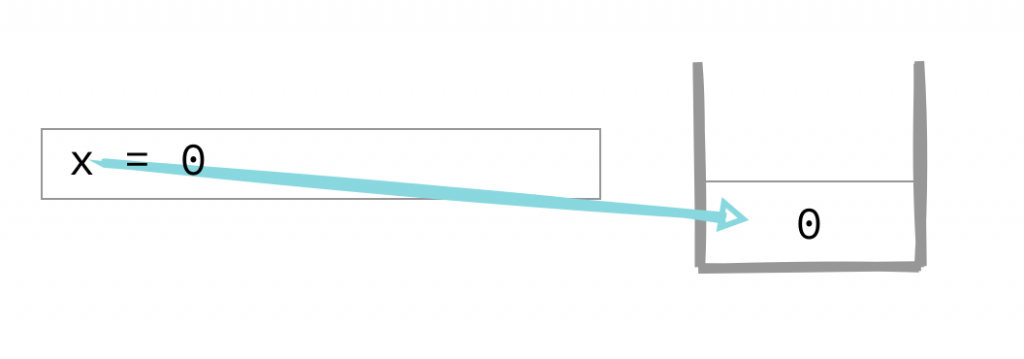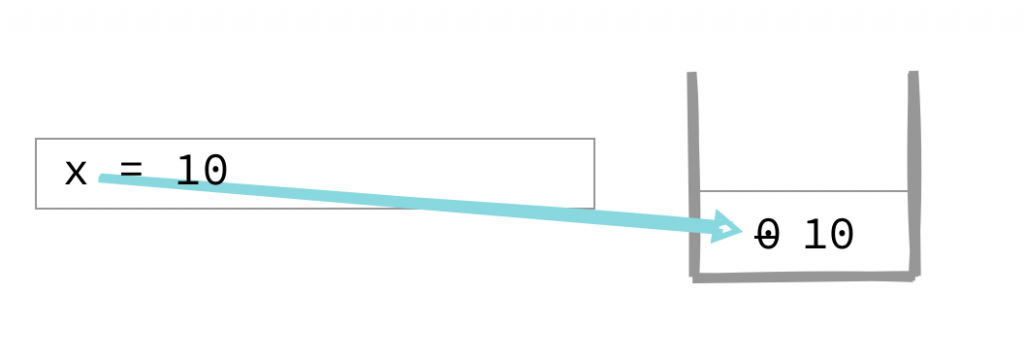## 不會變的…變數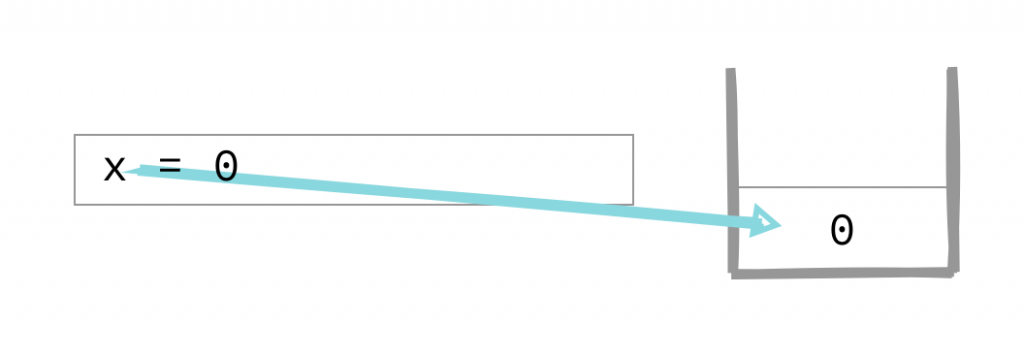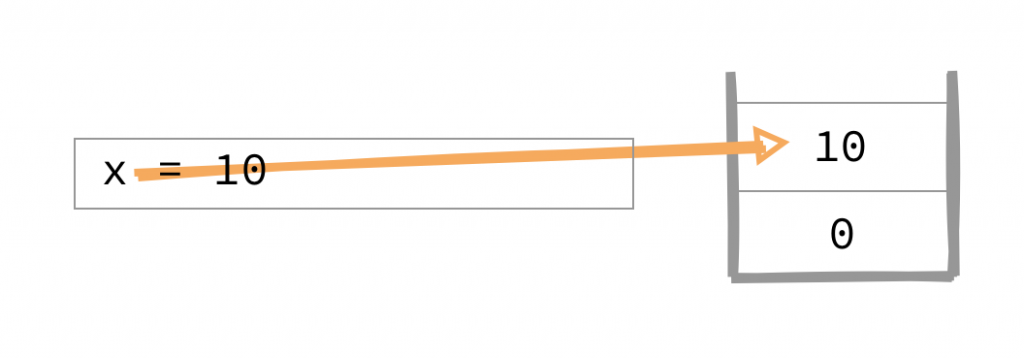## 函式內引用了外界變數

``````let x = 10

function foo(y) { return x + y }
foo(1) //=> 11

x = 99
foo(1) //=> 100
``````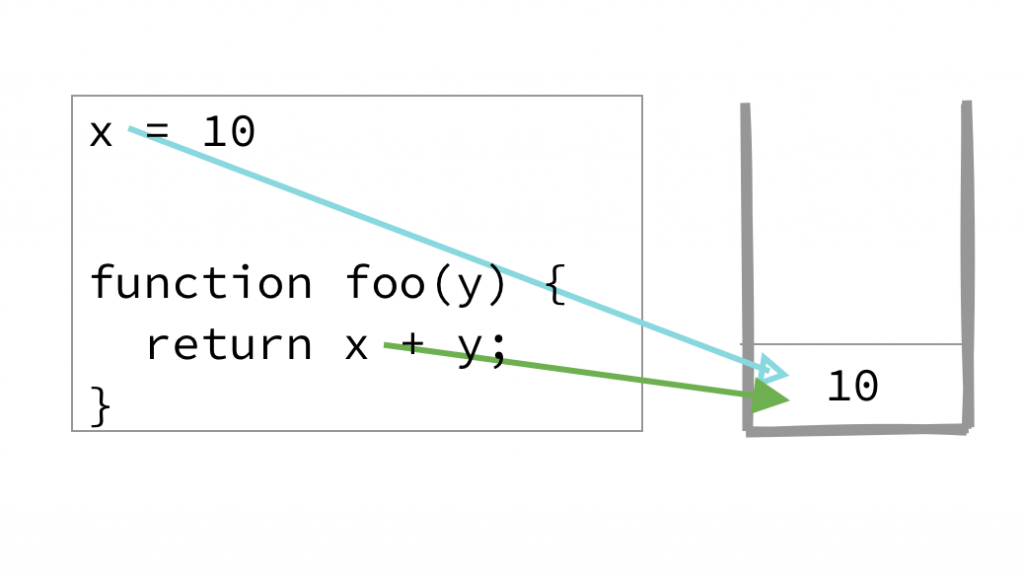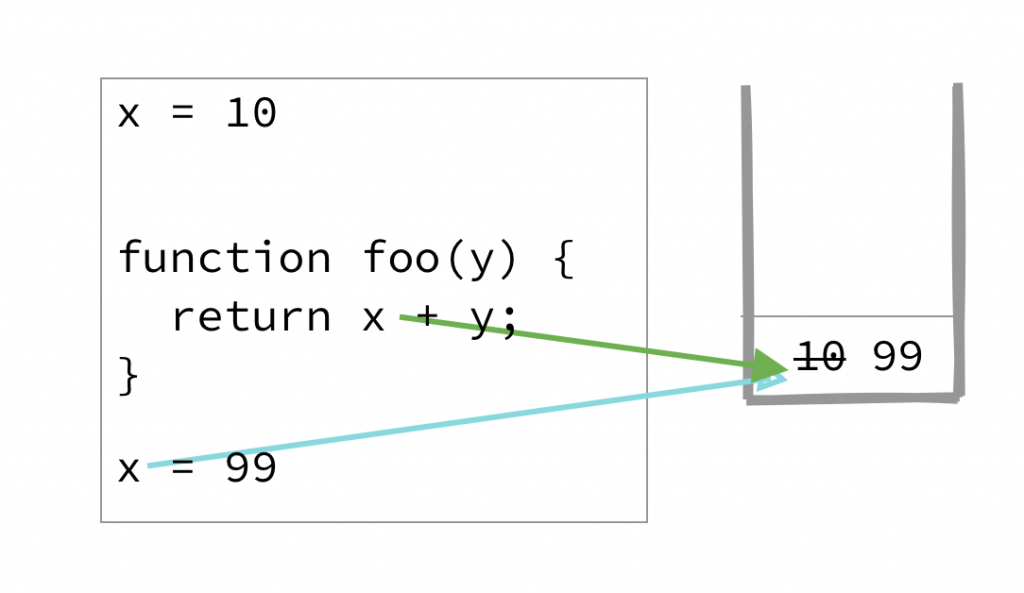``````# ruby 語法
x = 10

foo = lambda {|y| x + y}
foo.(1) #=> 11

x = 99
foo.(1) #=> 100
``````

(我想起之前想要在網頁裡用迴圈綁定事件，結果按按鈕都出現一樣的值的窘境)

## 把值…封裝起來

``````x = 10
foo = fn y -> x + y end
foo.(1) #=> 11

x = 99
foo.(1) #=> 11
``````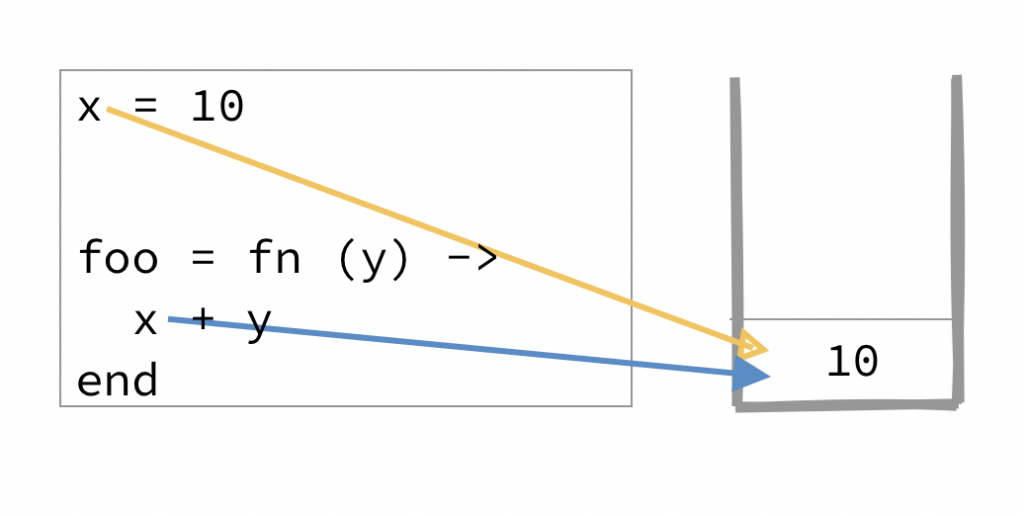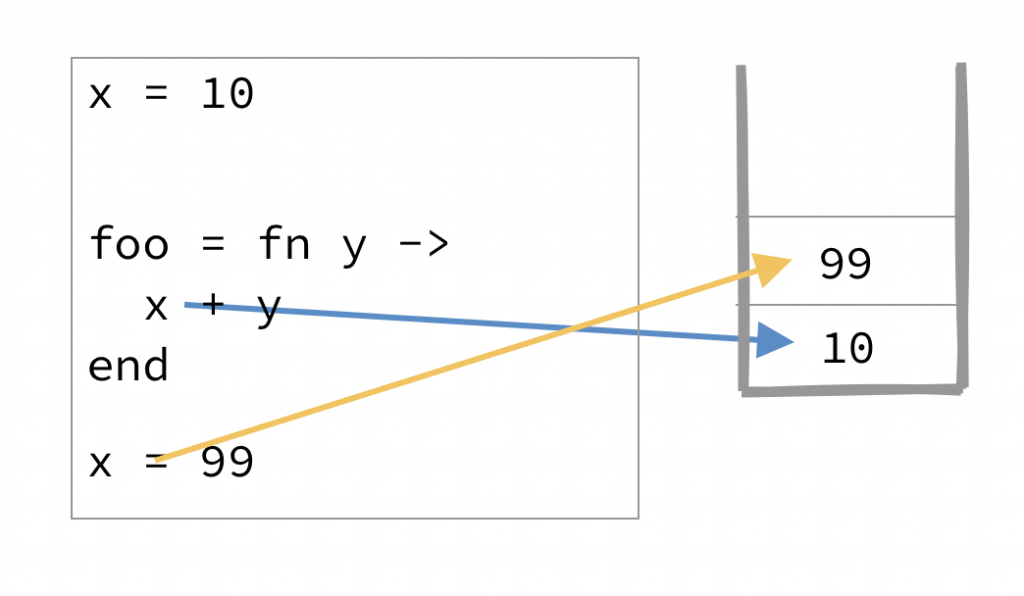## 怎麼在其它環境模仿這個行為

``````let x = 10

let bar = function(x) {
return function(y) { return x + y }
}
let foo = bar(x)
foo(1); //=> 11

x = 99
foo(1) //=> 11
``````

## 取捨

「所以，在這些語言…嗯…國度裡，等號就是變數綁定嗎？」

[to be continue]